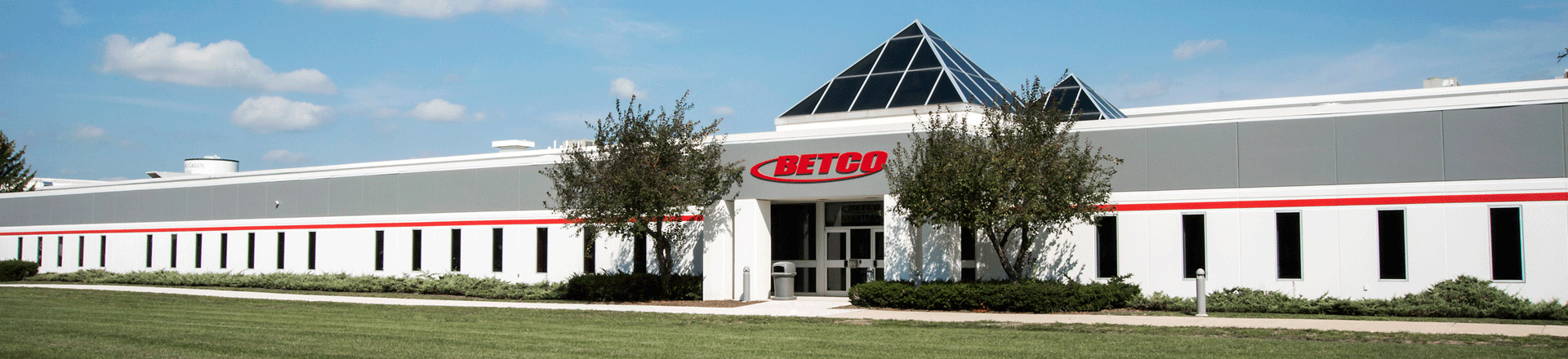Blog

Home > MyBetco > Blog

## Everything You Ever Wanted to Know About Manually Mixing Chemicals

Oct 29, 2019Introduction
Manually mixing concentrated chemicals with water—also known as the “glug glug” method—is a practice that still permeates the commercial cleaning and maintenance industry today. Every facility has unique needs, but improper dilution of chemicals in any environment not only results in ineffective and inconsistent outcomes, but furthermore is costly and even dangerous.

Improper dilution of chemicals can lead to unsightly streaks on glass, sticky residue on carpet (which causes rapid resoiling), or permanent damage of sensitive surfaces like aluminum and marble. It could also expose patrons of a restaurant to foodborne illness, increase slip and fall accidents in the aisles of a grocery store, or leave patients in a hospital vulnerable to infection.

Clearly, it is of critical importance to properly mix concentrated cleaning solutions, and the first step in doing so is to consult the product label for the dilution ratio. If a product is multi-purpose, the label will often indicate different dilution ratios for different applications, so it is important to select the appropriate ratio for the task at hand.

Dilution Ratios and Ounces per Gallon
Dilution ratios are written as 2 numbers separated by a colon. The number to the left of the colon represents the amount of concentrated chemical, while the number to the right of the colon represents the amount of water. For example:In this example, the dilution ratio can be verbally expressed as 1 part chemical to 32 parts water.

In place of or in addition to the dilution ratio, the label may supply the number of ounces of concentrated chemical per gallon of water.

If given the dilution ratio only, ounces per gallon may be calculated by dividing 128 (the number of ounces in a gallon) by the amount of water (the number to the right of the colon). In continuing with our previous example of a 1:32 dilution ratio:

128 (ounces in a gallon) ÷ 32 = 4 ounces per gallon of water

If given ounces per gallon only, the dilution ratio may be calculated by dividing 128 by the recommended number of ounces per gallon. The quotient is the number of parts water to 1 part chemical, and as such goes to the right of the colon. For example, if a label indicates 4 ounces per gallon:

128 (ounces in a gallon) ÷ 4 = 32, or a dilution ratio of 1:32

Other Units of Volume
If you would like to calculate ounces of concentrated chemical per a different amount of water, simply swap 128 for the appropriate number of ounces:
• 10 gallons = 1280 ounces
• 5 gallons = 640 ounces
• 1 gallon = 128 ounces
• 1 quart = 32 ounces
• 1 pint = 16 ounces
• 1 cup = 8 ounces

For example, if you want to know how much concentrated chemical to use with a quart of water, and the dilution ratio is 1:32:

32 (ounces in a quart) ÷ 32 = 1 ounce per quart of water

The Metric System
If calculating milliliters per liter from dilution ratio or dilution ratio from milliliters per liter, the same logic holds. Using a dilution ratio of 1:32:

1000 (milliliters in 1 liter) ÷ 32 = 31.25 milliliters per liter

1000 (milliliters in 1 liter) ÷ 31.25 = 32, or a dilution ratio of 1:32

If conversion between metric and imperial is necessary, here are some common metric volume equivalents:
• 1 ounce = 29.6 milliliters
• 1 cup = 236.6 milliliters
• 1 pint = 473.2 milliliters
• 1 quart = 946.4 milliliters (or 0.9464 liters)
• 1 gallon = 3.8 liters (or 3785.4 milliliters)
• 5 gallons = 18.9 liters (or 18927.1 milliliters)
• 10 gallons = 37.9 liters (or 37854.1 milliliters)
• 33.8 ounces = 1 liter (or 1000 milliliters)

Real-World Applications
Most of the time, the ready-to-use solution will go into a receptacle with an actual volume that is slightly greater than the advertised volume. For example, a 5-gallon pail really holds approximately 5.75 gallons. For this reason, it is often easiest and most expedient to use 5 gallons of water plus the appropriate amount of concentrated chemical, even though this results in more than 5 gallons. Using our familiar 1:32 dilution ratio example:

4 (ounces of concentrated chemical per gallon of water) x 5 (gallons of water) = 20 ounces of concentrated chemical

20 (ounces of concentrated chemical) + 5 (gallons of water) = 5 gallons, 20 ounces of ready-to-use solution

The total volume of 5 gallons, 20 ounces will usually not be a problem.

Cost per Diluted Gallon
Finally, it may be useful to calculate cost per diluted gallon.

For this calculation, the parts concentrated chemical and water expressed in the dilution ratio must be added together. For our 1:32 dilution ratio:

1 + 32 = 33 parts

Then divide the concentrated chemical’s cost per gallon by the total parts. For example, if the cost is \$10.00 for 1 gallon of concentrated chemical:

\$10.00 ÷ 33 (total parts) = \$0.30 per diluted gallon

Safety
When manually mixing chemical, it is important to always add water before you add the concentrated chemical in order to minimize chemical splash and foam. As with any chemical, always read the Safety Data Sheet before use and be sure to wear the proper PPE, such as gloves and eye protection.

If you would like to avoid the hassle and safety risks associated with manually mixing chemicals, Betco® offers several closed dilution control systems that consistently provide the correct dilution for cleaning staff. Learn more here.